# 100 100 200 200 300 300 400 400

• Slides: 69\$100 \$100 \$200 \$200 \$300 \$300 \$400 \$400 \$500 \$500Graph It!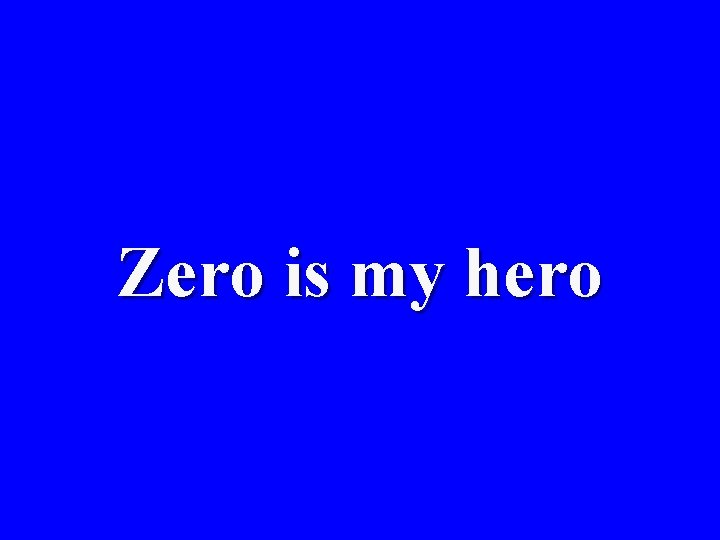Zero is my heroYou’re such a square…root!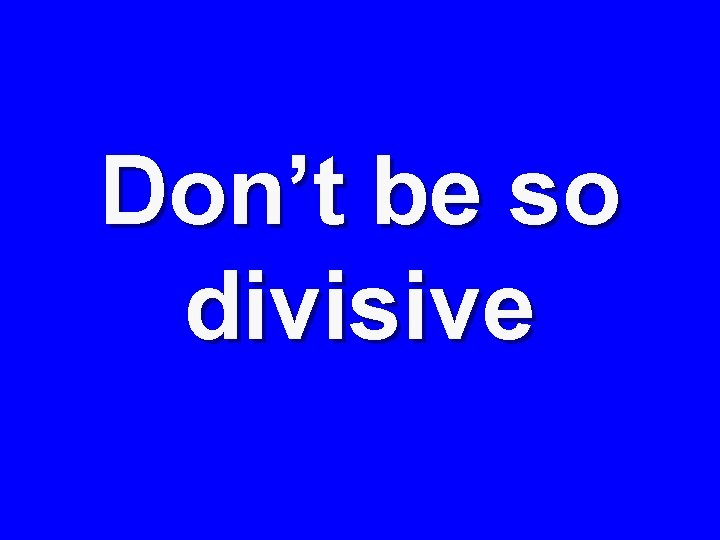Don’t be so divisive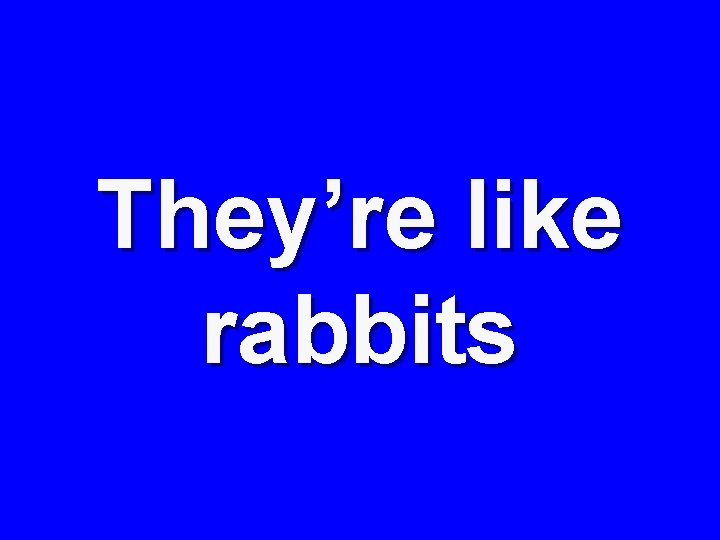They’re like rabbitsGraph It! Zero is my hero! \$100 \$100 \$200 \$200 \$300 \$300 \$400 \$400 \$500 \$500 Your such Don’t be so They’re like a divisive rabbits Square…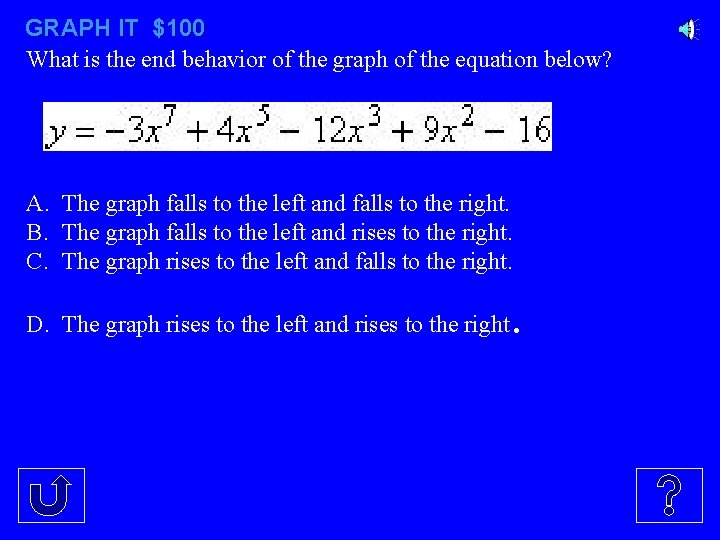GRAPH IT \$100 What is the end behavior of the graph of the equation below? A. The graph falls to the left and falls to the right. B. The graph falls to the left and rises to the right. C. The graph rises to the left and falls to the right. D. The graph rises to the left and rises to the right .GRAPH IT - - \$200 Which of these shows the graph of the equation A B C D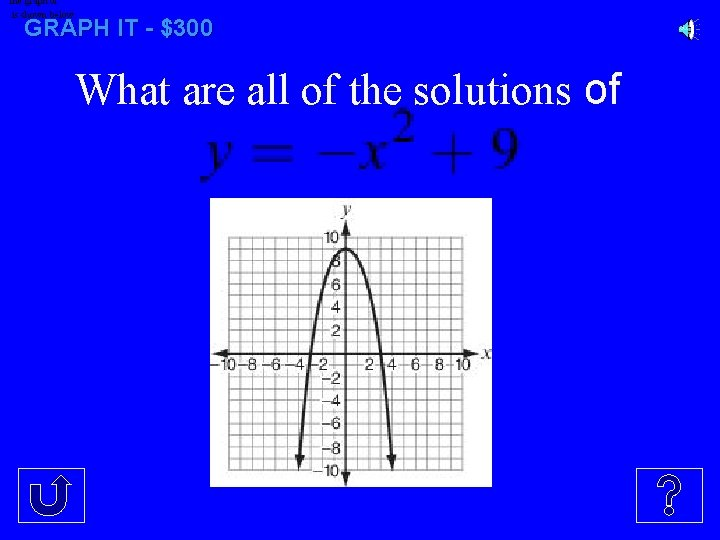The graph of is shown below. GRAPH IT - \$300 What are all of the solutions ofGRAPH IT - \$400 The factors of the polynomial expression for this graph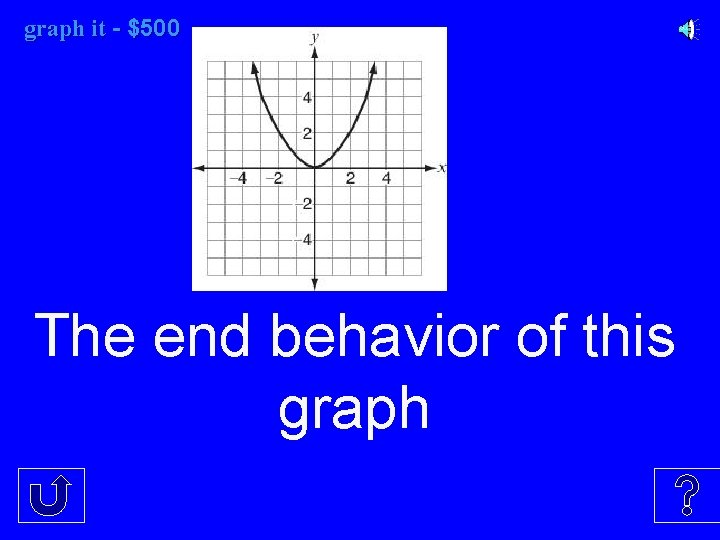graph it it - \$500 The end behavior of this graphZeros - \$100 The zeros of this graph.Zeros \$200 The zeros of this graph.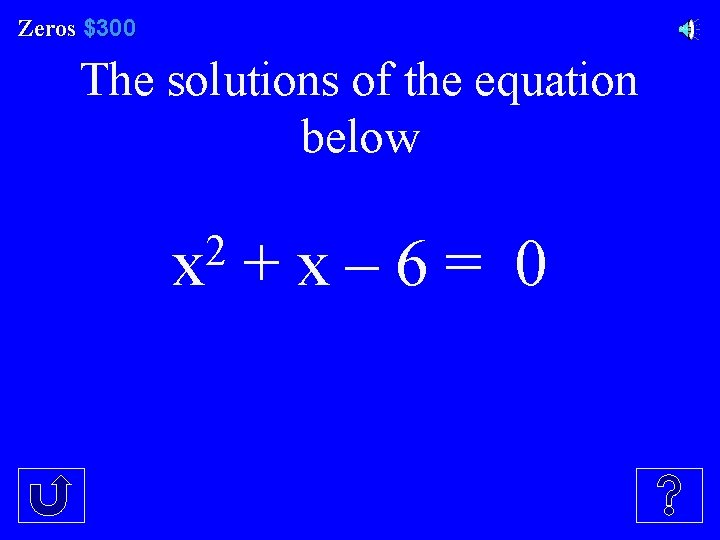Zeros \$300 The solutions of the equation below 2 x + x – 6 = 0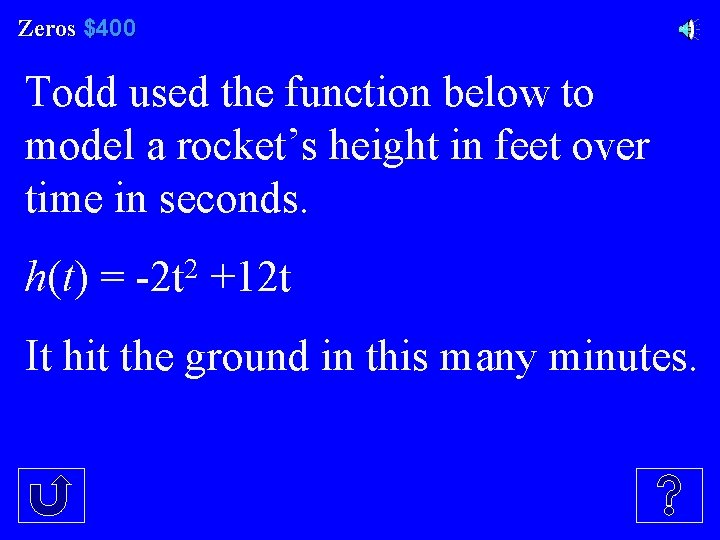Zeros \$400 Todd used the function below to model a rocket’s height in feet over time in seconds. h(t) = -2 t 2 +12 t It hit the ground in this many minutes.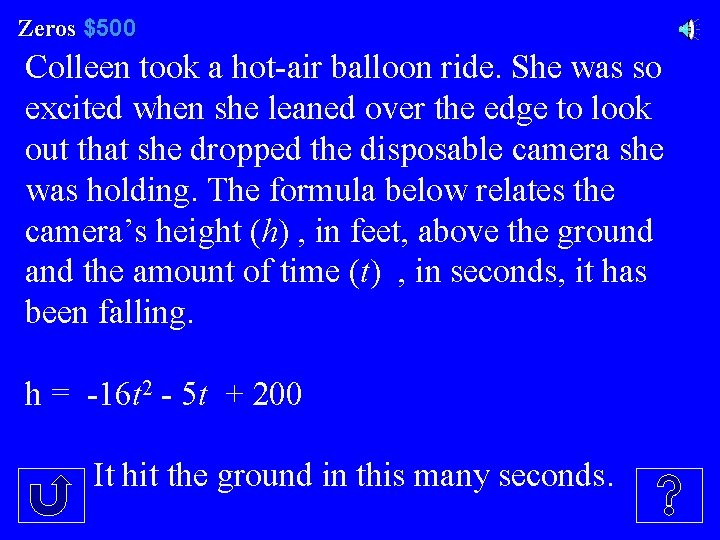Zeros \$500 Colleen took a hot-air balloon ride. She was so excited when she leaned over the edge to look out that she dropped the disposable camera she was holding. The formula below relates the camera’s height (h) , in feet, above the ground and the amount of time (t) , in seconds, it has been falling. h = -16 t 2 - 5 t + 200 It hit the ground in this many seconds.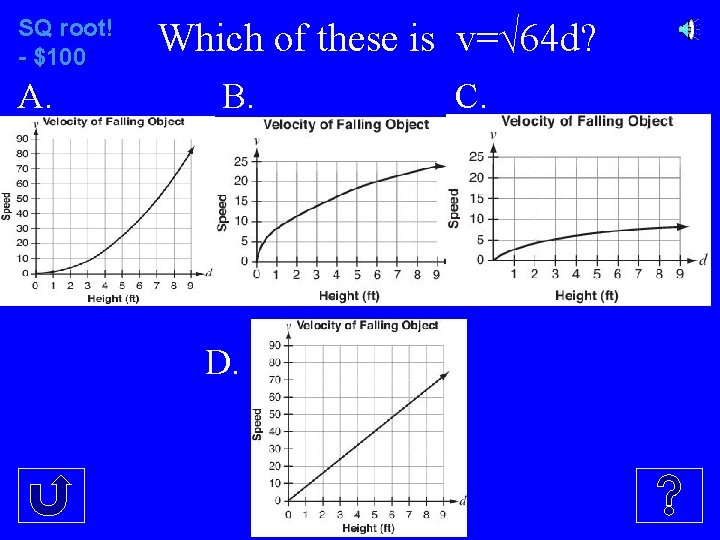SQ root! - \$100 A. Which of these is v=√ 64 d? B. D. C.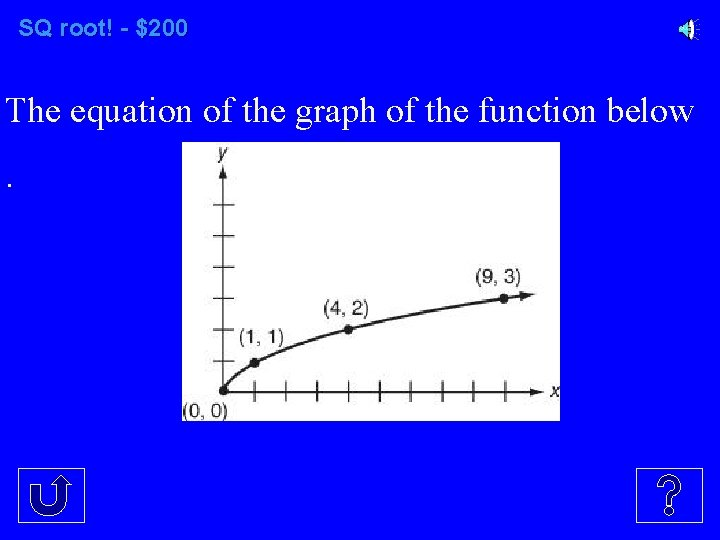SQ root! - \$200 The equation of the graph of the function below.SQ root! ! - \$300 The graph of the function f(x) = 1. 22√x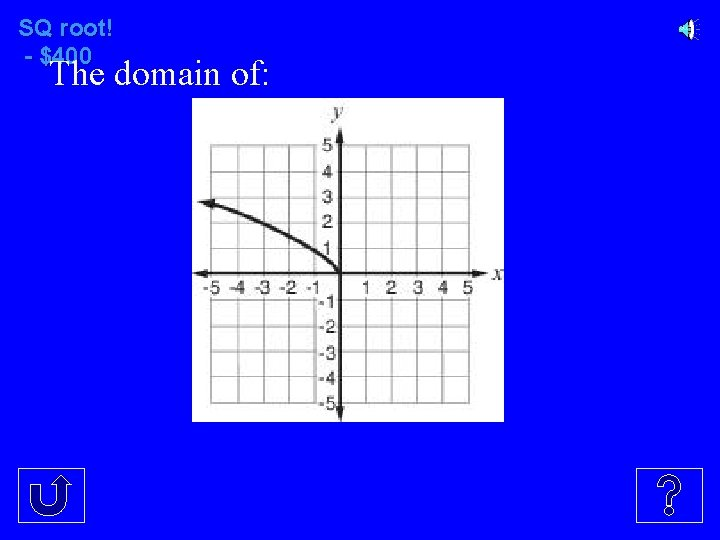SQ root! - \$400 The domain of: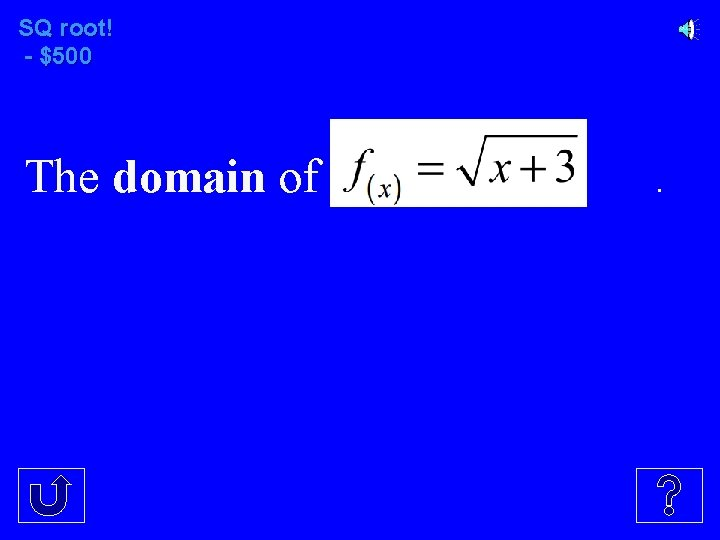SQ root! - \$500 The domain of .Don’t be so divisive - \$100Don’t be so divisive - \$200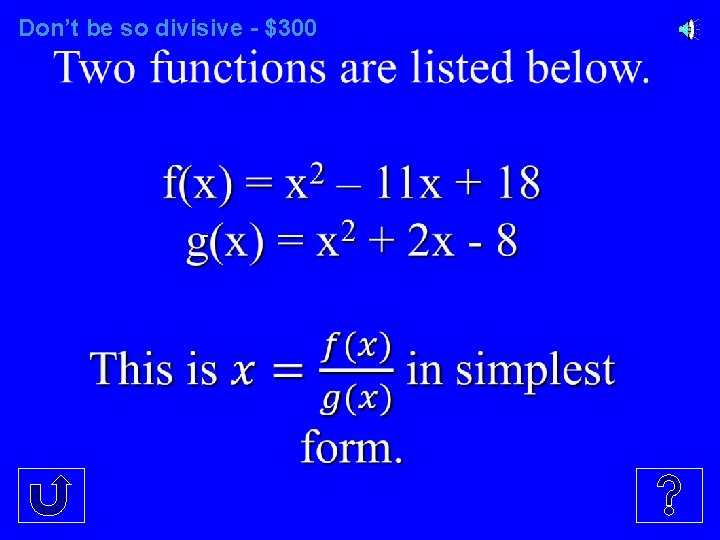Don’t be so divisive - \$300Don’t be so divisive - \$400Don’t be so divisive - \$500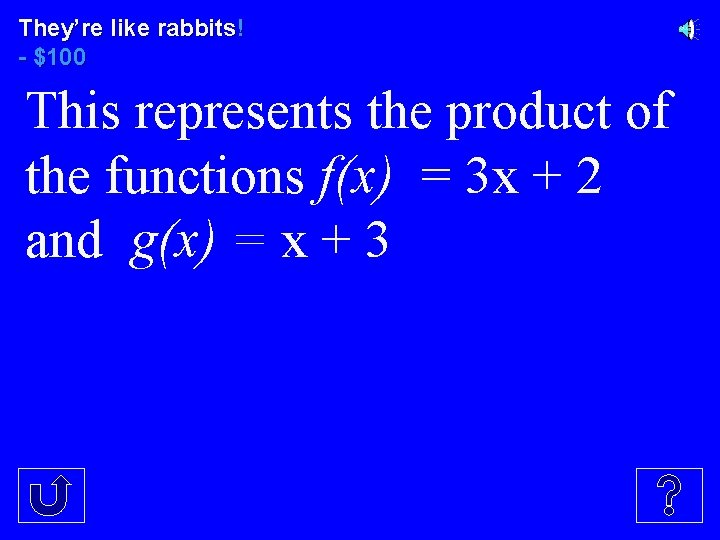They’re like rabbits! - \$100 This represents the product of the functions f(x) = 3 x + 2 and g(x) = x + 3They’re like rabbits! - \$200 Olga is using this model to multiply 4 y 2 by y – 3. This term goes in the empty box.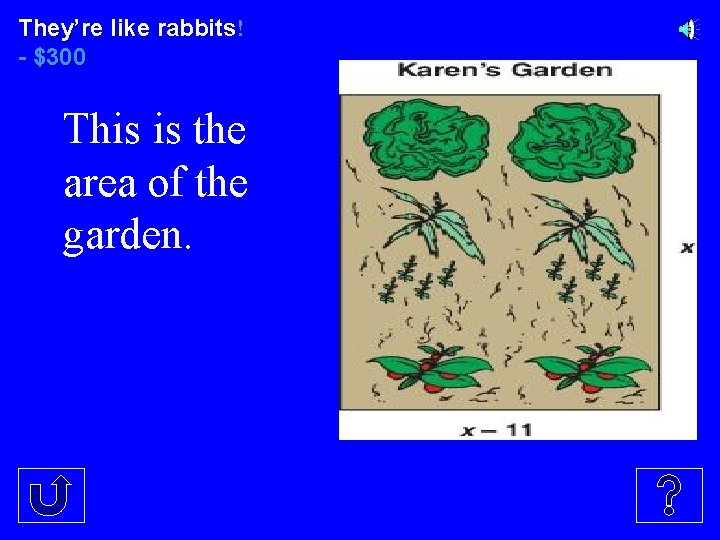They’re like rabbits! - \$300 This is the area of the garden.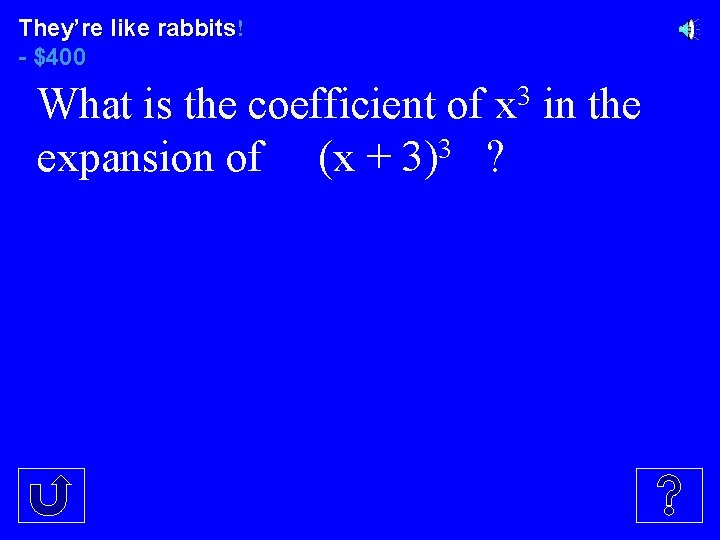They’re like rabbits! - \$400 What is the coefficient of x 3 in the expansion of (x + 3)3 ?They’re like rabbits! - \$500 2 This is the coefficient of x in 4 the expansion of (3 x + 4)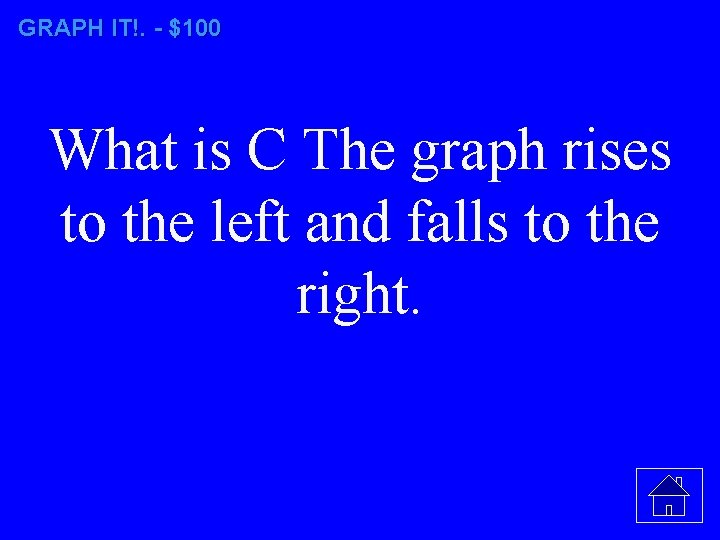GRAPH IT!. - \$100 What is C The graph rises to the left and falls to the right.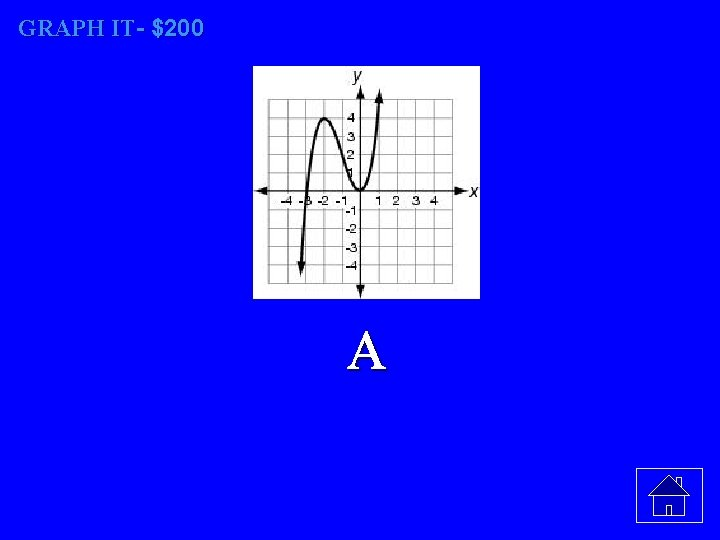GRAPH IT- \$200 A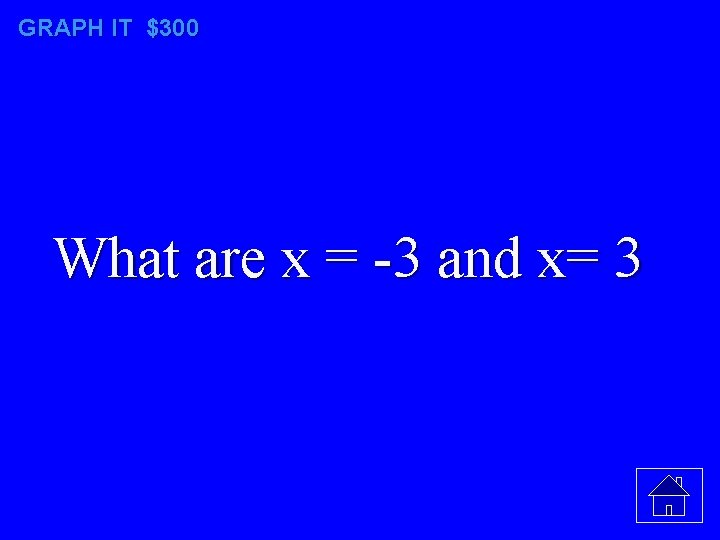GRAPH IT \$300 What are x = -3 and x= 3Props - \$400 What are the x-intercepts of a graph are -3, -1 and 4 ?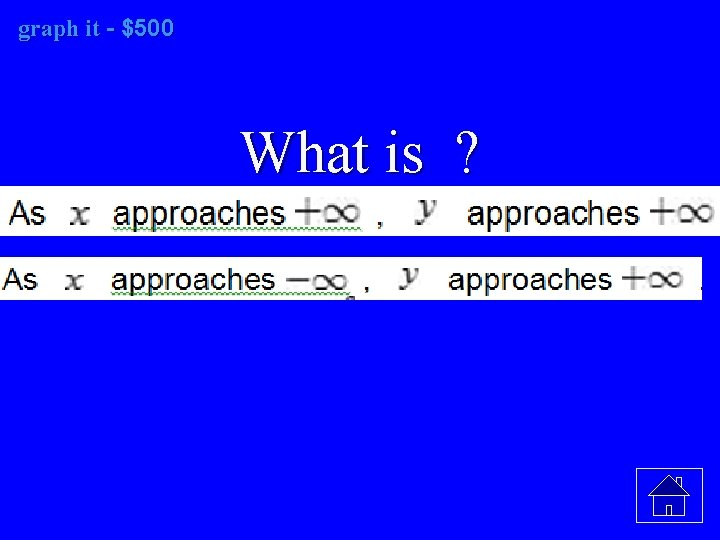graph it it - \$500 What is ?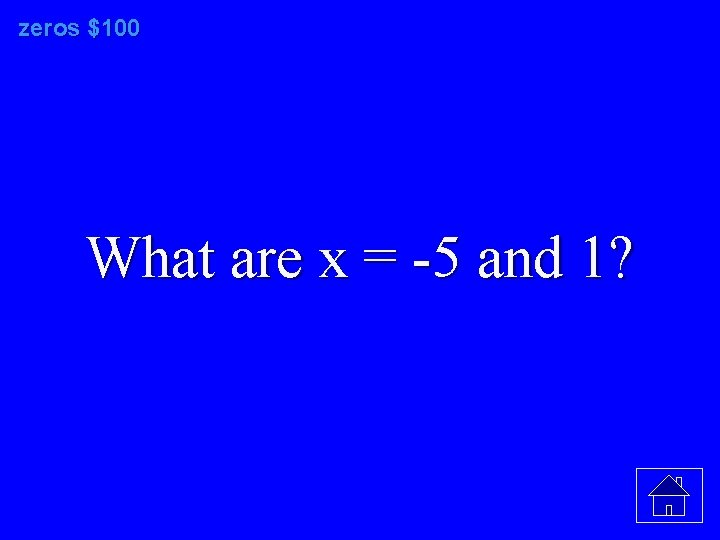zeros \$100 What are x = -5 and 1?zeros \$200 What are x = -5 and 7?zeros \$300 What are x = -3 and 2?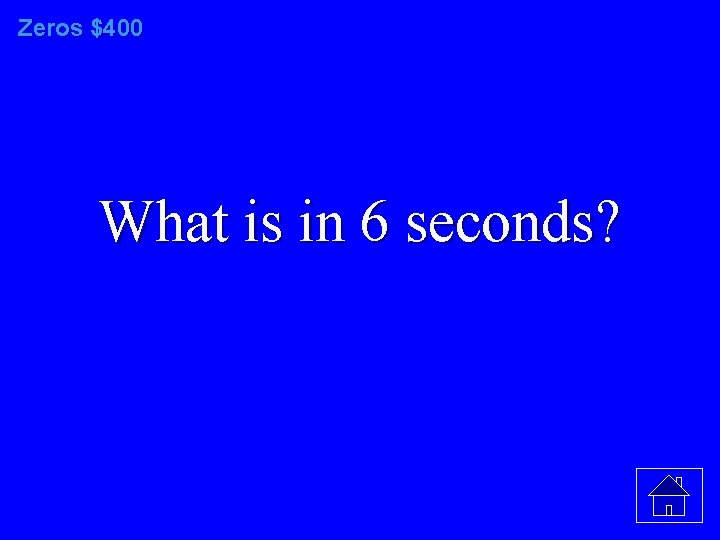Zeros \$400 What is in 6 seconds?zeros \$500 What is 3. 38 seconds What is ?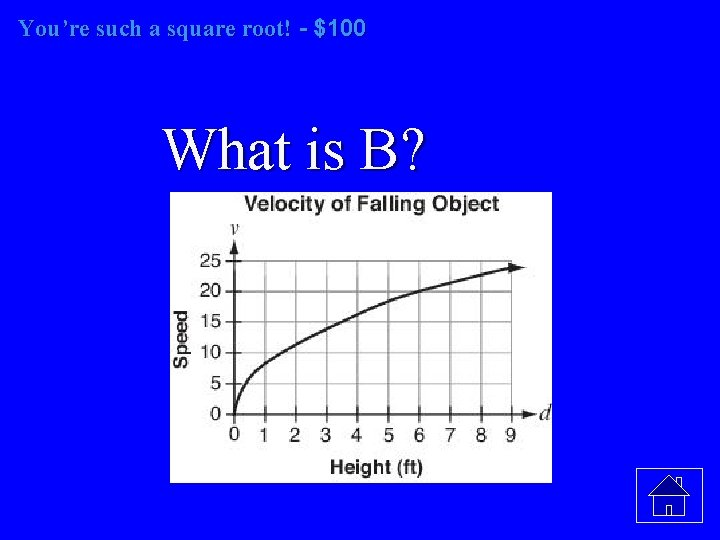You’re such a square root! - \$100 What is B?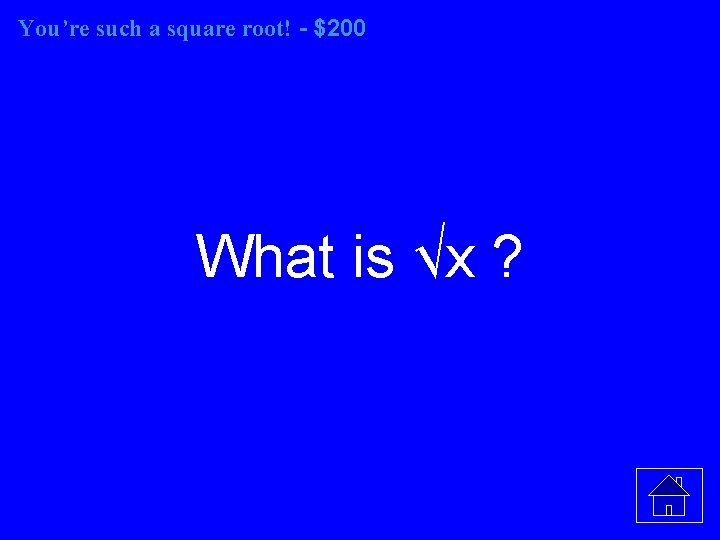You’re such a square root! - \$200 What is √x ?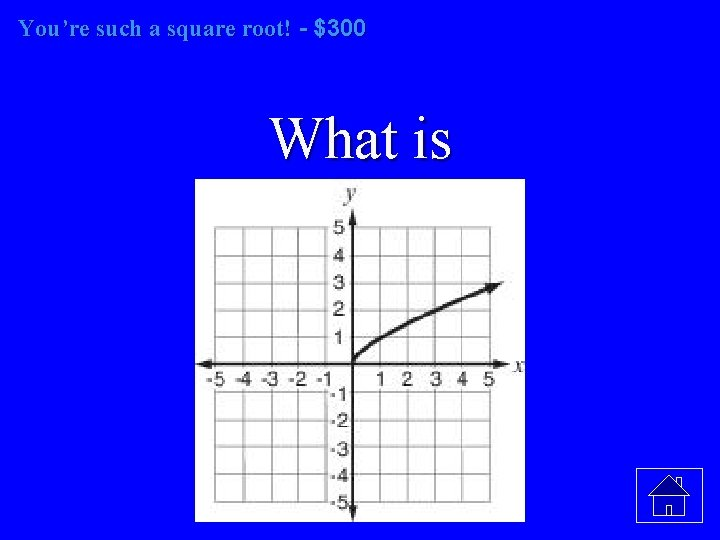You’re such a square root! - \$300 What is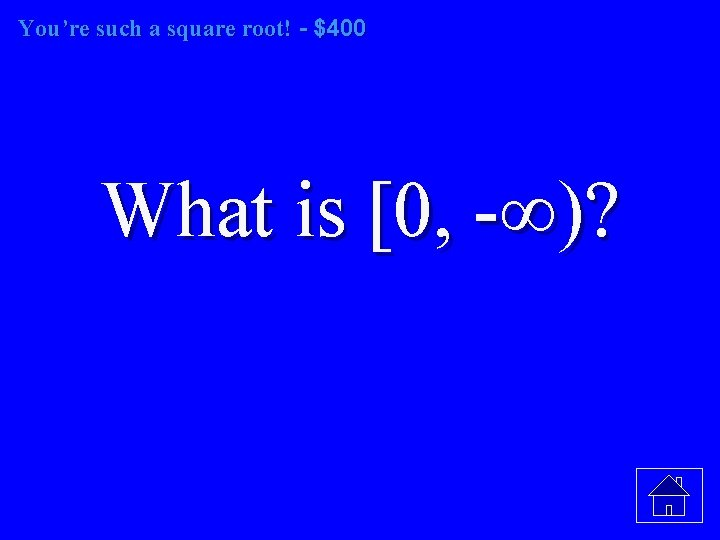You’re such a square root! - \$400 What is [0, -∞)?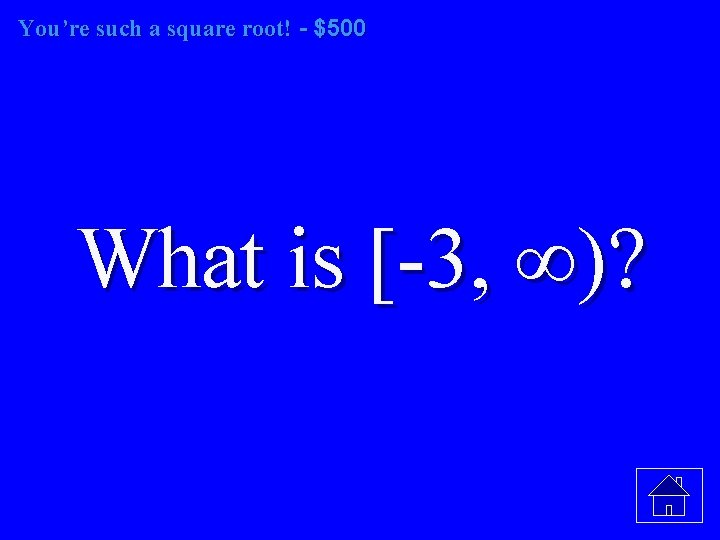You’re such a square root! - \$500 What is [-3, ∞)?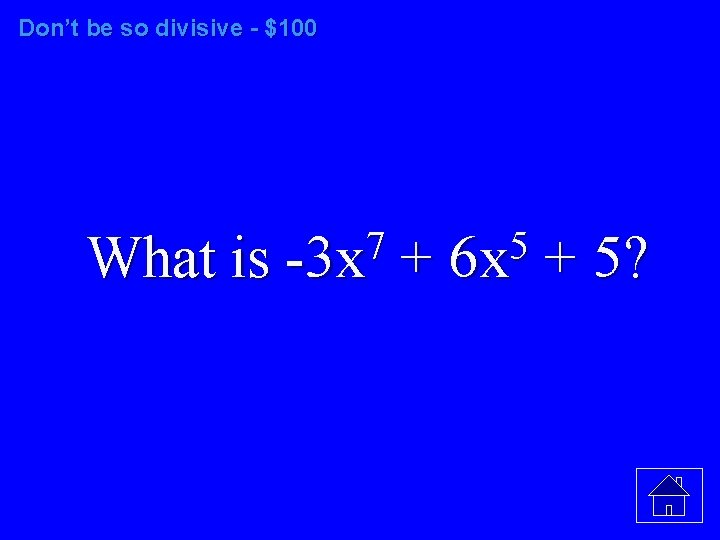Don’t be so divisive - \$100 7 5 What is -3 x + 6 x + 5?Don’t be so divisive \$200 What is ?Don’t be so divisive - \$300 What is ?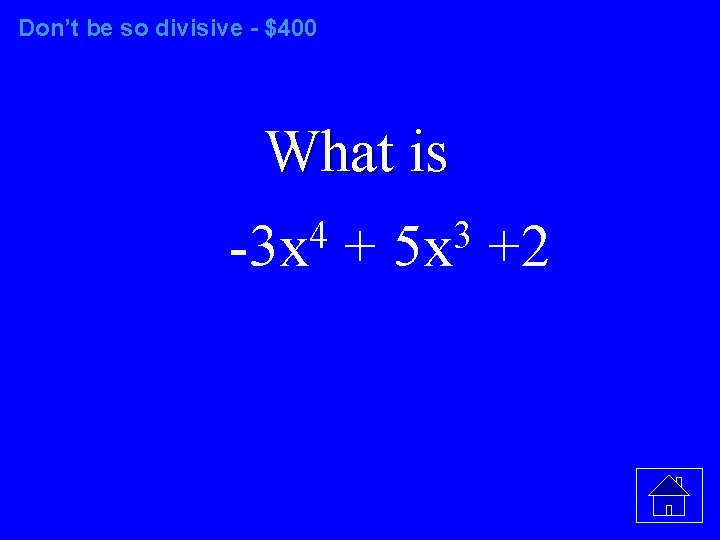Don’t be so divisive - \$400 What is 4 3 -3 x + 5 x +2Don’t be so divisive - \$500 What is ?They’re like rabbits- \$100 2 What is 3 x + 11 x + 6 ?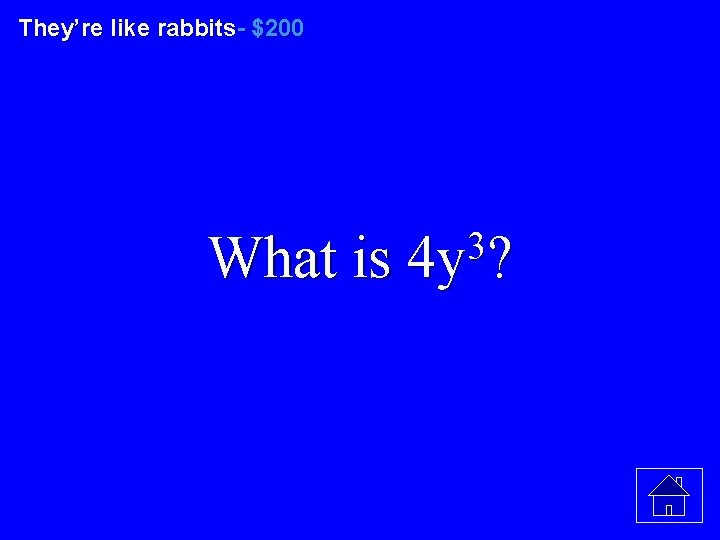They’re like rabbits- \$200 3 What is 4 y ?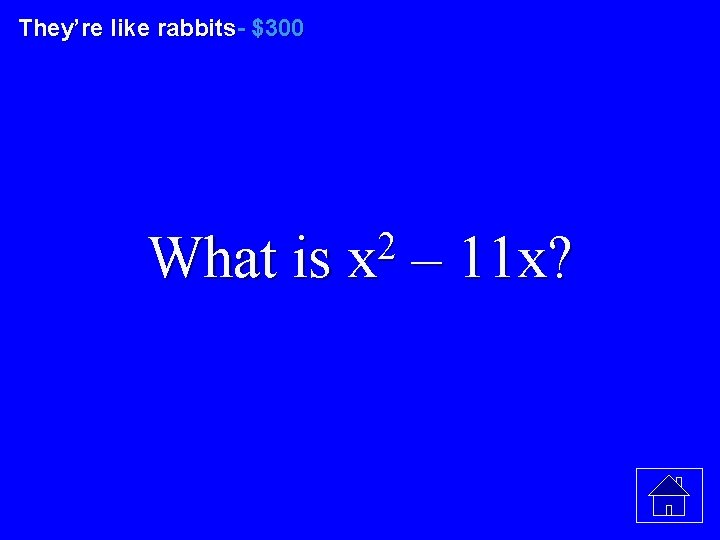They’re like rabbits- \$300 2 What is x – 11 x?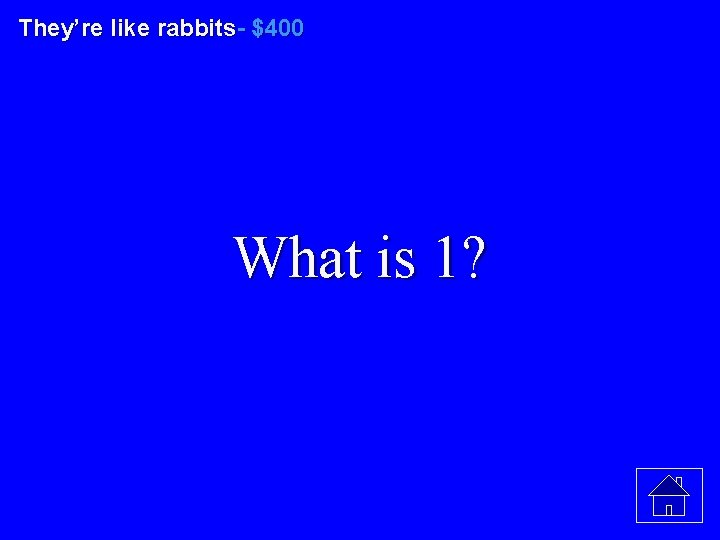They’re like rabbits- \$400 What is 1?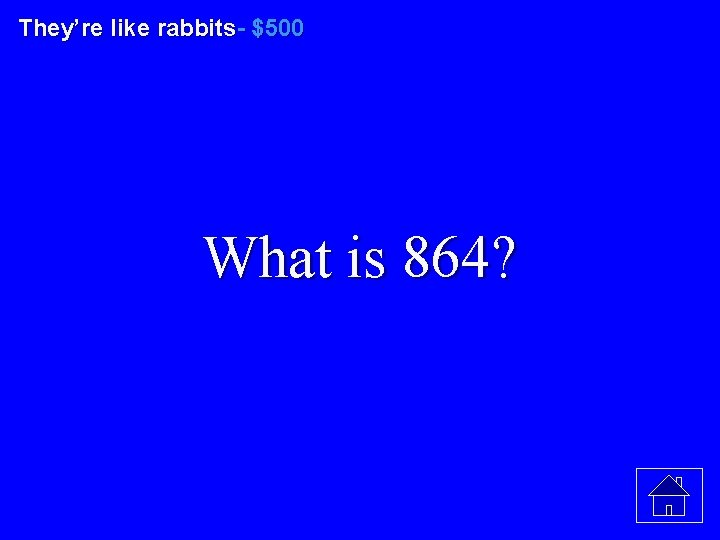They’re like rabbits- \$500 What is 864?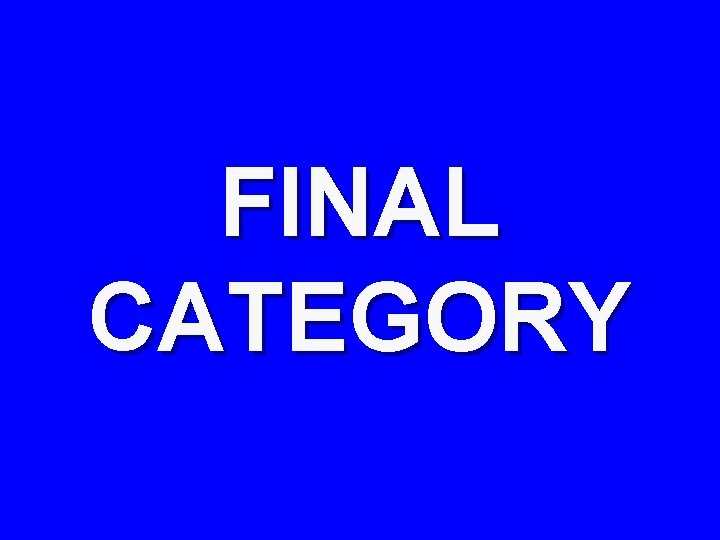FINAL CATEGORYFINAL CATEGORY Pascal’s Love TriangleFINAL CATEGORY 3 This is the coefficient of x in the expansion of 4 (x + 4) ?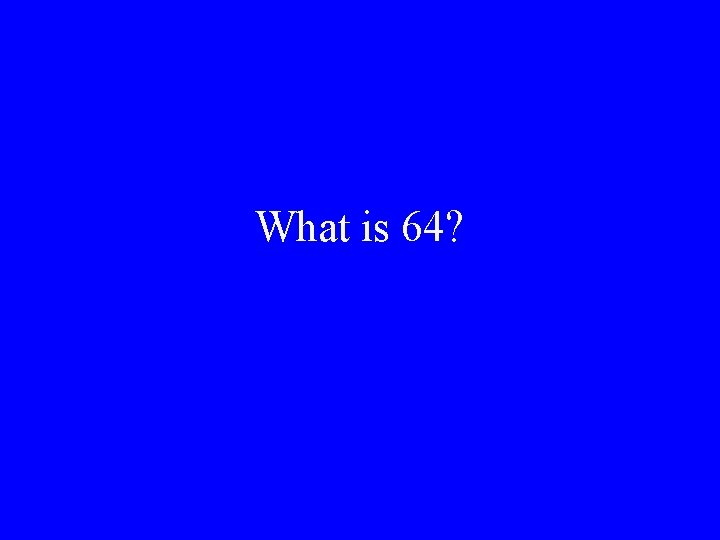What is 64?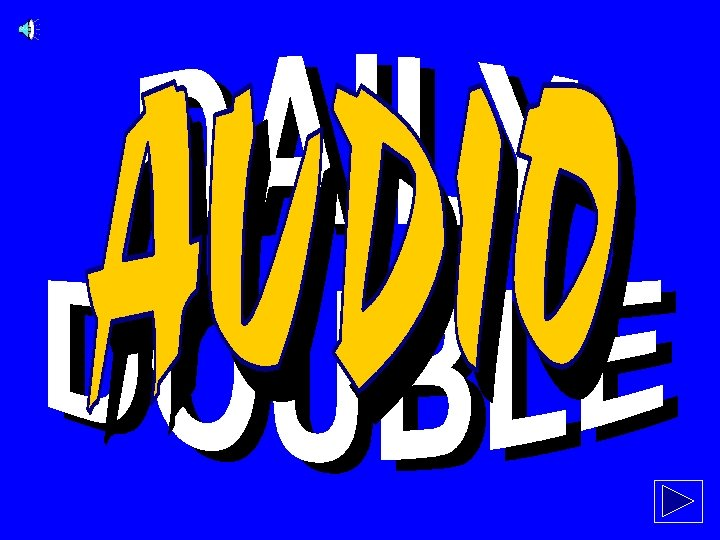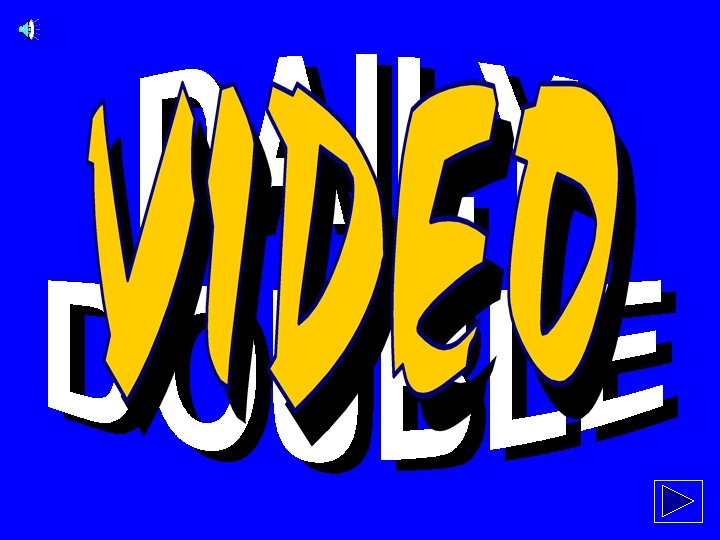JEOPARDY! Slide Show Notes • • The font for the question & answer slides is “Enchanted; ” a copy of this font in located in the “REAL Jeopardy Template” folder. (This font will need to be installed in the C: /WINDOWS/FONTS folder of the computer running the show. ) In order to keep all of the sounds and fonts together, copy the entire “REAL Jeopardy Template” folder. To change the categories: – – • 1. Go to “Edit” and “Replace…” 2. In the Find box, type CATEGORY 1 (all caps) 3. In the Replace box, type the category in all caps (for example, PRESIDENTS) 4. Click Replace All. . . To use the Daily Double: – 1. Choose which dollar values to set as Daily Double – 2. Link that dollar value to one of the DD slides – 3. Link the arrow on the DD slide to the correct question slide (so dollar/category match)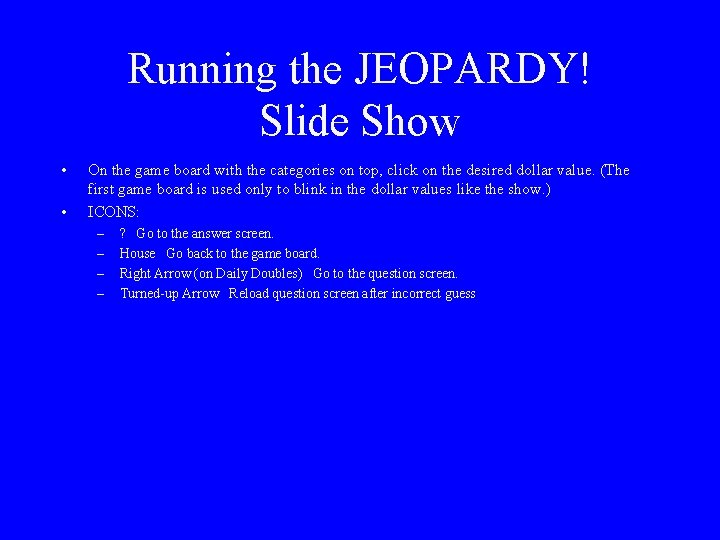Running the JEOPARDY! Slide Show • • On the game board with the categories on top, click on the desired dollar value. (The first game board is used only to blink in the dollar values like the show. ) ICONS: – – ? Go to the answer screen. House Go back to the game board. Right Arrow (on Daily Doubles) Go to the question screen. Turned-up Arrow Reload question screen after incorrect guess